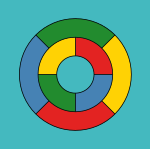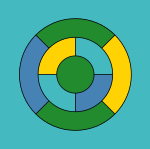# Four color theorem facts for kids

Kids Encyclopedia Facts

The four color theorem is a theorem of mathematics. It says that in any plane surface with regions in it (people think of them as maps), the regions can be colored with no more than four colors. Two regions that have a common border must not get the same color. They are called adjacent (next to each other) if they share a segment of the border, not just a point.

This was the first theorem to be proved by a computer, in a proof by exhaustion. In proof by exhaustion, the conclusion is established by dividing it into cases, and proving each one separately. There may be a lot of cases. For example, the first proof of the four color theorem was a proof by exhaustion with 1,936 cases. This proof was controversial because most of the cases were checked by a computer program, not by hand. The shortest known proof of the four color theorem today still has over 600 cases.

Even though the problem was first presented as a problem to color political maps of countries, mapmakers are not very interested in it. According to an article by the math historian Kenneth May (Wilson 2002, 2), “Maps utilizing only four colors are rare, and those that do usually require only three. Books on cartography and the history of map making do not mention the four-color property.”

Many simpler maps can be colored using three colors. The fourth color is needed for some maps, such as one in which one region is surrounded by an odd number of others, which touch each other in a cycle. One such example is given in the image. The five color theorem states that five colors are enough to color a map. It has a short, elementary proof and was proven in the late 19th century. (Heawood 1890) Proving that four colors are all that is needed turned out to be much more difficult. Many false proofs and false counterexamples have appeared since the first statement of the four color theorem in 1852.

## Exact formulation of the problem

Intuitively, the four color theorem can be stated as 'given any separation of a plane into contiguous regions, called a map, the regions can be colored using at most four colors so that no two regions which are adjacent have the same color'. To be able to correctly solve the problem, it is necessary to clarify some aspects: First, all points that belong to three or more countries must be ignored. Secondly, bizarre maps with regions of finite area and infinite perimeter can require more than four colors.

For the purpose of the theorem every "country" has to be a simply connected region, or contiguous. In the real world, this is not true: Alaska as part of the United States, Nakhchivan as part of Azerbaijan, and Kaliningrad as part of Russia are not contiguous. Because the territory of a particular country must be the same color, four colors may not be enough. For instance, consider a simplified map, such as the one shown on the left: In this map, the two regions labeled A belong to the same country, and must be the same color. This map then requires five colors, since the two A regions together are contiguous with four other regions, each of which is contiguous with all the others. If A had only three regions, six or more colors might be needed. In this way, it is possible to make maps that need an arbitrarily high number of colors. A similar construction also applies if a single color is used for all bodies of water, as is usual on real maps.

An easier to state version of the theorem uses graph theory. The set of regions of a map can be represented more abstractly as an undirected graph that has a vertex for each region and an edge for every pair of regions that share a boundary segment. This graph is planar: it can be drawn in the plane without crossings by placing each vertex at an arbitrarily chosen location within the region to which it corresponds, and by drawing the edges as curves that lead without crossing within each region from the vertex location to each shared boundary point of the region. Conversely any planar graph can be formed from a map in this way. In graph-theoretic terminology, the four-color theorem states that the vertices of every planar graph can be colored with at most four colors so that no two adjacent vertices have the same color, or for short, "every planar graph is four-colorable" ( Thomas 1998, p. 849; Wilson 2002).

## History

The first person to name the problem was Francis Guthrie, in 1852. He was a law student in England, at the time. He found that he needed at least four colors to color a map of the counties of England. Augustus de Morgan first discussed the problem, in a letter he wrote to Rowan Hamliton in August 1852. In the letter, de Morgan asks whether four colors are really enough to color a map, such that countries that are next to each other get different colors.

English mathematician Arthur Cayley presented the problem to the mathematical society in London, in 1878. Within a year, Alfred Kempe found what looked like a proof of the problem. Eleven years later, in 1890, Percy Heawood showed that Alfred's proof was wrong. Peter Guthrie Tait presented another attempt at a proof in 1880. It took eleven years to show that Tait's proof did not work either. In 1891, Julius Petersen could show this. When he falsified Cayley's proof, Kempe also showed a proof for a problem he called Five color theorem. The theorem says that any such map can be colored with no more than five colors. There are two restrictions: First, any country is contiguous, there are no exclaves. The second restriction is that countries need to have a common border; if they only touch in a point, they can be colored with the same color. Even though Kempe's proof was wrong, he used some of the ideas which would later permit a correct proof.

In the 1960s and 1970s, Heinrich Heesch developed a first sketch of a proof by computer. Kenneth Appel and Wolfgang Haken improved this sketch in 1976 (Robertson and others 1996). They were able to reduce the number of cases that would need to be tested to 1936; a later version was made that relied on testing only 1476 cases. Each case needed to be tested by a computer (Appel & Haken 1977).

In 1996, Neil Robertson, Daniel Sanders, Paul Seymour and Robin Thomas improved the method, and reduced the number of cases to be tested to 663. Again, each case needed to be tested by computer.

In 2005, Georges Gonthier and Benjamin Werner developed a formal proof. This was an improvement, because it allowed to use theorem-proving software, for the first time. The software used is called Coq.

The four color theorem is the first big mathematical problem that was proved with the help of a computer. Because the proof cannot be done by a human, some mathematicians did not recognize it as correct. To verify the proof, it is necessary to rely on a correctly working software and hardware to validate the proof. Because the proof was done by computer, it is also not very elegant.

## Attempts that turned out to be wrong

The four color theorem has been notorious for attracting a large number of false proofs and disproofs in its long history. At first, The New York Times refused to report on the Appel–Haken proof. The newspaper did this as a matter of policy; it feared that the proof would be shown false like the ones before it (Wilson 2002, p. 209). Some proofs took a long time, till they could be falsified: For Kempe's and Tait's proofs falsifying them took over a decade.The map (left) has been colored with five colors, and it is necessary to change at least four of the ten regions to obtain a coloring with only four colors (right).

The simplest counterexamples generally try to create one region which touches all the others. This forces the remaining regions to be colored with only three colors. Because the four color theorem is true, this is always possible; however, because the person drawing the map is focused on the one large region, they do not notice that the remaining regions can in fact be colored with three colors.

This trick can be generalized: If the colors of some regions in a map are chosen beforehand, it becomes impossible to color the remaining regions in such a way that in total, only four colors are used. Someone verifying the counterexample may not think that it may be needed to change the color of these regions. This will make the counterexample look valid, even though it is not.

Perhaps one effect underlying this common misconception is the fact that the color restriction is not transitive: a region only has to be colored differently from regions it touches directly, not regions touching regions that it touches. If this were the restriction, planar graphs would require arbitrarily large numbers of colors.

Other false disproofs violate the assumptions of the theorem in unexpected ways, such as using a region that has multiple disconnected parts, or not allowing regions of the same color from touching at a point.

## Coloring political maps

In real life, many countries have exclaves or colonies. Since they belong to the country, they need to be colored with the same color as the parent country. This means that usually, more than four colors are needed to color such a map. When mathematicians talk about the graph associated with the problem, they say that is not planar. Even though it is easy to check if a graph is planar, finding the fewest number of colors needed to color it is very difficult. It is NP-complete, which is one of the most difficult problems that exist. The fewest number of colors needed to color a graph is known as its chromatic number. Many of the problems that happen when trying to solve the four color theorem are related to discrete mathematics. For this reason, methods from algebraic topology are often used.

## Extension to "non-flat" maps

The four color theorem requires the "map" to be on a flat surface, what mathematicians call a plane. In 1890, Percy John Heawood created what is called Heawood conjecture today: It asks the same question as the four color theorem, but for any topological object. As an example, a torus can be colored with at most seven colors. The Heawood conjecture gives a formula that works for all such objects, except the Klein bottle.

### Articles and Books

• .
• .